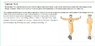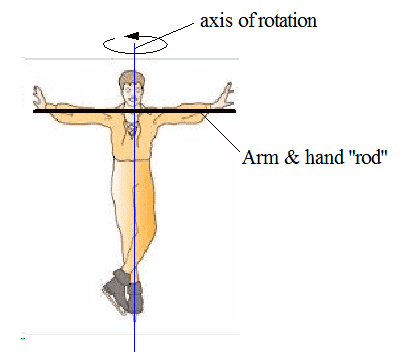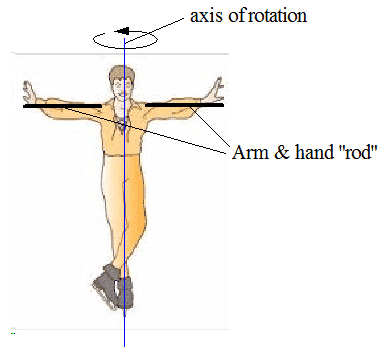# Moment of inertia calculation

## Homework Statement

Kindly see the screenshot attached below for the question.

I=1/3ML^2
1/12ML^2

## The Attempt at a Solution

In the solution to this question, the moment of inertia of the hands (when outstretched) is taken to be 1/12ML^2 (combined). I think that it should be 1/3ML^2 because the axis is near the shoulder. Also, wouldn't it make any difference if we are calculating the combined moment of the rods rather than calculating individually.

#### Attachments

•Screenshot_89.jpg
30.7 KB · Views: 403
It depends what you take as M and L. If you consider it as two end-pivoted rods I is 2*1/3ML2. If you consider it as one centre-pivoted rod of mass 2M and length 2L, I = 1/12*2M*(2L)2 =2/3ML2. As you are given the mass and length of the two outstretched arms together, you should use I = 1/12ml2

In the solution to this question, the moment of inertia of the hands (when outstretched) is taken to be 1/12ML^2 (combined). I think that it should be 1/3ML^2 because the axis is near the shoulder.
In their simplified model they are considering the "arm and hand" rod to be a single rod passing through the axis of rotation. The rotation axis is definitely not at the shoulder! It passes vertically though the center of the body.Also, wouldn't it make any difference if we are calculating the combined moment of the rods rather than calculating individually.
Moments of inertia sum algebraically, so no, if the rod is continuous.

What would make a difference is if you were to form a more complex model where each arm+had is considered as separate rod attached at the shoulders. The axis of rotation would still be through the center of the body, so you'd have to apply the parallel axis theorem to calculate the moment of inertia of the rods about an axis that is offset from the end of the rod.#### Attachments

•Delta2
1/12ML^2 (combined). I think that it should be 1/3ML^2
It's the same thing. Each arm (measured from the spine) is length L/2, mass m/2. Applying the 1/3 formula for a rod about its end point that gives 1/3(m/2)(L/2)2 = mL2/24 for each arm, and a total of mL2/12.